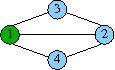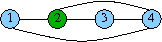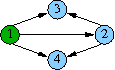TCS / Software / `bliss`

# bliss: A Tool for Computing Automorphism Groups and Canonical Labelings of Graphs

### Authors: Tommi Junttila and Petteri Kaski

bliss is an open source tool for computing automorphism groups and canonical forms of graphs. It has both a command line user interface as well as C++ and C programming language APIs.

## Graphs, Isomorphism, and Canonical Forms

Below we briefly formally describe what bliss does. For the algorithms and data structures of bliss, refer to the paper Engineering an efficient canonical labeling tool for large and sparse graphs. For more on the graph isomorphism problem, see e.g. wikipedia and the links there.

A colored graph is a triple G=(V,E,c) where V={1,2,...,N} is a finite set of vertices, E is a set of 2-element subsets of V called edges, and c:V→N is a function that associates to each vertex a nonnegative integer (a color). For example, identifying blue with 0 and green with 1, the graphs in Figure 1 are

G1 = ({1,2,3,4}, {{1,2},{1,3},{1,4},{2,3},{2,4}}, ⟨1→1,2→0,3→0,4→0⟩)

and

G2 = ({1,2,3,4}, {{1,2},{1,4},{2,3},{2,4},{3,4}}, ⟨1→0,2→1,3→0,4→0⟩).

Figure 1: Two colored graphs(a) G1 (b) G2
Let γ:V→V be a permutation of V. Denote by vγ the image of v∈V under γ. For a colored graph G=(V,E,c), define the colored graph

Gγ = (V, {{vγ,wγ} | {v,w}∈E}, cγ),

where cγ:V→N is defined for all v∈V by cγ(vγ)=c(v).

Two colored graphs, G1=(V,E1,c1) and G2=(V,E2,c2), are isomorphic if there exists a permutation γ:V→V such that G1γ=G2. Such a permutation γ is called an isomorphism of G1 onto G2. For example, there are two possible isomorphisms of G1 onto G2 in Figure 1, namely ⟨1→2,2→4,3→1,4→3⟩ and ⟨1→2,2→4,3→3,4→1⟩. We write G1≅G2 to indicate that G1 and G2 are isomorphic.

An automorphism of a colored graph is an isomorphism of the colored graph onto itself. The set of all automorphisms of a colored graph G forms a permutation group where the group operation is the functional composition of permutations. This permutation group is called the automorphism group of G and is denoted by Aut(G). A set of generators for Aut(G) is a set S⊆Aut(G) such that every non-identity permutation in Aut(G) can be obtained by composing elements of S. For example, {⟨1→1,2→2,3→4,4→3⟩} is a set of generators for

Aut(G1)={⟨1→1,2→2,3→3,4→4⟩,⟨1→1,2→2,3→4,4→3⟩}

in Figure 1.

A function ρ from colored graphs to colored graphs is a canonical representative map if the following two conditions hold:

• the representative of a graph is isomorphic to the graph, i.e., for all colored graphs G it holds that ρ(G)≅G, and
• the representatives of two graphs are the same if and only if the graphs are isomorphic, i.e., for all colored graphs G1,G2 it holds that G1≅G2 iff ρ(G1)=ρ(G2).
A canonical labeling of G (under ρ) is an isomorphism of G onto its canonical representative ρ(G). Given a colored graph G as input, the software tool bliss computes the canonical representative ρ(G) and an associated canonical labeling for it. Also computed is a set of generators for Aut(G).

### Directed Graphs

The software tool bliss can also handle directed graphs. Formally, a directed colored graph is a triple G=(V,E,c), where V and c are the vertex set and the color function as in the undirected case above but E⊆V×V is now a set directed edges. As an example, identifying white with 0 and grey with 1, the directed graph in Figure 2 is

({1,2,3,4}, {(1,2),(1,3),(1,4),(2,3),(2,4)}, ⟨1→1,2→0,3→0,4→0⟩)

Given a permutation γ:V→V of V and a directed colored graph G=(V,E,c), define the directed colored graph

Gγ=(V, {(vγ,wγ) | (v,w)∈E}, cγ),

where cγ:V→N is defined for all v∈V by cγ(vγ)=c(v). The definitions of automorhism, isomorphism, generator sets, and canonical labelings for directed colored graphs are the same as for undirected colored graphs.
Figure 2: A directed graph### A Note of Caution

In general, the computed canonical form depends on the version and applied options of bliss. Therefore, if you are building and storing a library of graphs in canonical form—for example, to classify some combinatorial structures up to isomorphism—be sure to use the same version of bliss with the same options all the time. In principle, the canonical forms should not depend on the computer platform in which bliss is compiled and run, but this has not been extensively tested in practice.

## Tools that use bliss

• igraph, a free software package for creating and manipulating undirected and directed graphs, developed at The Computational Neuroscience Group in the KFKI Research Institute for Particle and Nuclear Physics of the Hungarian Academy of Sciences.

## Related software and some other relevant links

[TCS main] [Contact Info] [Personnel] [Research] [Publications] [Software] [Studies] [News Archive] [Links]
Latest update: 01 September 2015. Tommi Junttila